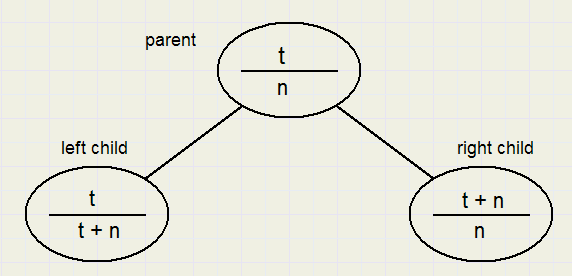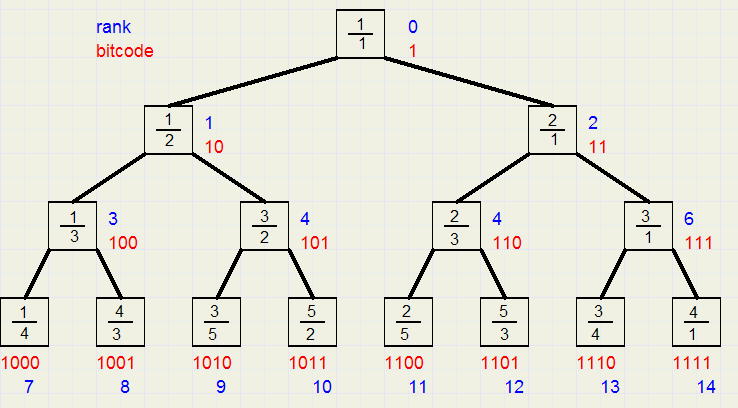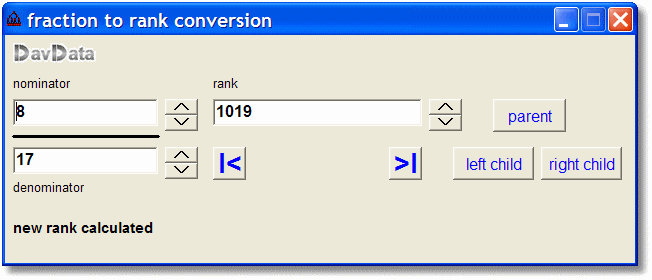the Rank of a Fractiondownloadprogramdownload completeDelphi-7 project

Theory

It is possible to assign a unique sequential number (called rank) to a fraction.
This is the trick:
if t / n is a non reducable fraction, then 2 other fractions my be derived , called the left- and the right child.
See picture belowStarting with 1/1 at the top, all possible fractions may be covered in this way.
Important is that
- no fraction is omitted
- no fraction will appear more than once
- fractions are irreducable (have no common factors)
Above rules are easy to prove, using contradiction.
Also, the process is very similar to the Euclidian algorithm to calculate the GCD of two numbers.

Picture below shows the top partEach fraction is assigned a bit code.
Starting with code 1 at the top, the left child adds a "0" to the code of the parent,
the right child adds a "1" .
To start with rank 0 for the top fraction, the rank of a fraction is simply it's code - 1.

In the picture above the bit code is listed in red, the rank in blue.

The ProgramA program has been written for the calculation of the rank of a fraction and vice versa.
Edit fields for the nominator, denominator and the rank allow for data entry.
When one field is changed, the other are adjusted.
Speedbuttons allow for sequential increment or decrement of each field.
Also it it possible to navigate through the tree with the parent and child buttons.